Courses

# SSC CPO Mock Test (GI & Reas) 4

## 50 Questions MCQ Test SSC CPO & Constable - Mock Tests & Previous Year Papers | SSC CPO Mock Test (GI & Reas) 4

Description
This mock test of SSC CPO Mock Test (GI & Reas) 4 for SSC helps you for every SSC entrance exam. This contains 50 Multiple Choice Questions for SSC SSC CPO Mock Test (GI & Reas) 4 (mcq) to study with solutions a complete question bank. The solved questions answers in this SSC CPO Mock Test (GI & Reas) 4 quiz give you a good mix of easy questions and tough questions. SSC students definitely take this SSC CPO Mock Test (GI & Reas) 4 exercise for a better result in the exam. You can find other SSC CPO Mock Test (GI & Reas) 4 extra questions, long questions & short questions for SSC on EduRev as well by searching above.
QUESTION: 1

Solution:
QUESTION: 2

Solution:
QUESTION: 3

### Re-arrange the given words in alphabetical order and tick the one that comes in the middle.

Solution:
QUESTION: 4
Choose the numeral pair which is different from others .
Solution: 25 - 54 This is the only pair in which the two numbers have no common factor.
QUESTION: 5
Choose the word which is least like the other words in the group .
Solution:
QUESTION: 6
Choose the group of letters which is different from others.
Solution: This is the only group UPKEA containing three vowels.
QUESTION: 7
Complete the analogous pair.
Nurture : Neglect : : Denigrate : ?
Solution:
QUESTION: 8
Complete the analogous pair.
Earth : Sun : : Moon : ?
Solution:
QUESTION: 9
Complete the analogous pair.
Oasis : Sand : : Island : ?
Solution:
QUESTION: 10
Complete the analogous pair.
Ship : Sea : : Camel :?
Solution:
QUESTION: 11
Study the following and answer the question based on it.
1. A,B,C,D,E,F and G are sitting on a wall and they all facing to north.
2. C is at the right of D.
3. B is at the end and E is his neighbour.
4. G is sitting between E and F.
5. D is third from southen end.
Who is sitting at the right of D?
Solution:
QUESTION: 12
Find the number of triangles and squares in the given figure.
Solution:
QUESTION: 13
In the following alphabetical series, which letter will be 3rd form the right of 5th letter from the left side?
A B C D E F G H I J K L M N O P Q R S T U V W X Y Z
Solution:
QUESTION: 14
One morning after sunrise Vikram and Shailesh were standing in a lawn with their back towards each other. Vikram's shadow fell exactly towards his left-hand side. Which direction Shailesh was facing?
Solution:
QUESTION: 15
Group the following figures into three classes on the basis of identical properties.
Solution: (1,4), (2,3) and (5,6) are three different pairs of identical figures.
QUESTION: 16

Find the missing character.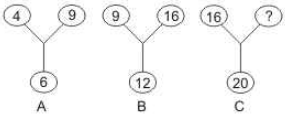Solution:

The square of the number at the bottom is equal to the product of the two upper numbers. Thus,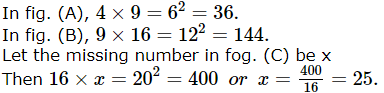QUESTION: 17
Find the missing character.
Solution:
QUESTION: 18

Find the missing character.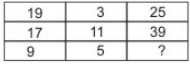Solution: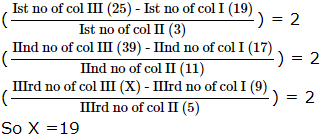QUESTION: 19

Choose the odd one out.

Solution:
QUESTION: 20

Choose the odd one out.

Solution:
QUESTION: 21
How many such pairs of letters are there in the word CLEARING each of which has two letters between them in the word and also in the alphabet?
Solution:
QUESTION: 22
If RASCAL is coded as QZRBZK then how will SOLDER be coded?
Solution:
QUESTION: 23
Which of the following diagrams indicates the best relation between travellers, train and bus?
Solution:
QUESTION: 24

select the correct combination of mathematical signs to replace * sign to balance the given equation.
6*1*6*4*2*6

Solution:
QUESTION: 25
Which of the following combination of circles best represents Athlets, Sprinters and Marathon runners?
Solution:
QUESTION: 26

If + means - , - means ×, × means ÷ and ÷ means + then
15 × 3 ÷ 15 + 5 - 2 = ?

Solution:

15 ÷ 3 + 15 - 5 × 2 = 5 + 15 - 5 × 2 = 5 + 15 - 10 = 10

QUESTION: 27
Complete the analogous pair.
125 : 17 18 21 :: 458 : ?
Solution:
QUESTION: 28
Choose the one which is different from the rest:
Solution:
QUESTION: 29
Choose the odd one out.
Solution:
QUESTION: 30
Choose the odd one out.
Solution:
QUESTION: 31
In this letter series, some of the letters are missing. Choose the correct letter given below -
aa _ ab _ _ aaa _ a
Solution:
QUESTION: 32
In this letter series, some of the letters are missing. Choose the correct letter given below -
_ bbca _ bcca _ ac _ a _ cb
Solution:
QUESTION: 33
Find the missing number/letters in the series.
625, 5, 125, 25, 25, ?, 5
Solution:
QUESTION: 34
Find the wrong term in the letter-number series given below :
G4T, J10R, M20P, P43N, S90L
Solution:
QUESTION: 35
Find out the term in the number series which is wrong.
5, 27, 61, 122, 213, 340, 509
Solution: The terms of the series are (23 - 3), (33 - 3), (43 - 3), (53 - 3), (63 - 3), (73 - 3), (83 -3).
So, 27 is wrong and must be replaced (33 - 3) i.e. 24
QUESTION: 36
Choose the correct alternative that will continue the same pattern and fill in the blank.
9, 11, 20, 31, (....), 82
Solution:
QUESTION: 37
Below is given statement followed by two assumptions numbered I and II. You have to consider the statement and the following assumptions and decide which of the assumptions is implicit in the statement :
Statement:
Government should deploy the Army atleast this year for the rehabilitation of people affected by cyclone because cyclone visits suddenly.
Assumptions:
I. The Army should be deployed for all such sudden incidents.
II. Some precautionary plan is being made to prevent destruction caused by cyclone.
Solution:
QUESTION: 38
Below is given statement followed by two conclusions numbered I and II. You have to consider the statement and the following conclusions and decide which of the conclusions is follows in the statement :
Statement :
All men should have equal opportunities but all did not have the same capacity. Some have the ability to earn more. Every one should use his ability for the benefit of the society.
Conclusions :
I. All men are not equal in all respects
II. One can use one's talents
Solution:
QUESTION: 39
If 'spoon' is called 'plate', 'plate' is called knife', 'knife' is called 'jug', 'jug' is called 'glass', 'glass' is called 'saucer' and 'saucer' is called 'spoon', by what do we cut fruit?
Solution:
QUESTION: 40
If Thursday was the day after the day before yesterday five days ago, what is the least number of days ago when Sunday was three days before the day after tomorrow ?
Solution: Day after the day before yesterday is yesterday.
Now, five days ago, yesterday was Thursday.
So, five days ago, it was Friday.
.'. Today is Wednesday.
Now, three days before the day after tomorrow is yesterday.
Now, it is on Monday that we say 'Yesterday was Sunday'.
QUESTION: 41
In each of the following questions, four groups of three words each are given. Only one group in each does not share the underlying principle that combines the words. Find that group:
Solution:
QUESTION: 42
Choose one word which cannot be made from the letters of the given word
UNCONSCIOUS
Solution:
QUESTION: 43

In the following questions, select a figure from amongst the four alternatives, which when placed in the blank space of fig. (X) would complete the pattern.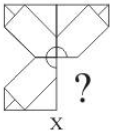Solution:
QUESTION: 44

In each question below is given a group of letters followed by four combinations of digits numbered (A), (B), (C) and (D). You have to find put which of the combinations correctly represents the group of letters based on the following coding system and mark the number of that combination as the answer. If none of the four combinations correctly represents the group of letters, mark (E) i.e. 'None of these' as the answer.
Letter/Symbol : M = S @ P ∆ D V *
Digits :                3  9 6  2  8  7 5  4  1
Conditions :
(i) If the first digit is odd and last digit is even, the codes for the first and the last digits are to be interchanged.
(ii) If the first as well as the last digit is even, both are to be coded by the code for last digit.
(iii) If the first as well as the last digit is odd, both are to be coded as 'X'.

285961

Solution:
QUESTION: 45

In each question below is given a group of letters followed by four combinations of digits numbered (A), (B), (C) and (D). You have to find put which of the combinations correctly represents the group of letters based on the following coding system and mark the number of that combination as the answer. If none of the four combinations correctly represents the group of letters, mark (E) i.e. 'None of these' as the answer.
Letter/Symbol : M = S @ P ∆ D V *
Digits :                3  9 6  2  8  7 5  4  1
Conditions :
(i) If the first digit is odd and last digit is even, the codes for the first and the last digits are to be interchanged.
(ii) If the first as well as the last digit is even, both are to be coded by the code for last digit.
(iii) If the first as well as the last digit is odd, both are to be coded as 'X'.

972486

Solution:
QUESTION: 46

Select the correct set of symbols which will fit in the given equation 5 0 3 5 = 20.

Solution:

5 - 0 + 3 × 5
= 5 + 15 = 20

QUESTION: 47

Select the correct mirror image of the figure (X) from amongst the given alternatives.

BOOK
(x)

Solution:
QUESTION: 48

Find out from amongst the four alternatives as to how the pattern would appear when the transparent sheet is folded at the dotted line.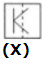Solution:
QUESTION: 49

In the following questions consists of five figures marked 1, 2, 3, 4 and 5 called the Problem Figures followed by five other figures marked A, B, C, D and E called the Answer Figures. Select a figure from the Answer Figures which will continue the same series as established by the five Problem Figures.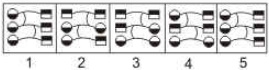Solution:
QUESTION: 50

In the questions, figure (X) is embedded in one of following figures. Find out the correct alternative.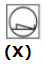Solution: